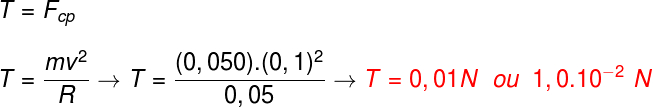Mechanics

# Centripetal force

Centripetal force is the resultant force that acts on a body and describes a motion in a circular path . It is responsible for changing the direction of the body’s velocity and, in addition, it always points to the center of the curves , so that the angle formed between this force and the velocity vector is always 90º.

## How to calculate centripetal force?

When a body performs a curvilinear motion, it is subject to a centripetal force . The centripetal force is equivalent to the resultant force on the body, in the radial direction (which points to the center of the curves). The formula used to calculate the centripetal force is shown below, check it out: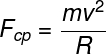CP – centripetal force (N)

m – mass (kg)

v – speed (m/s)

R – radius of the curve (m)

The centripetal force can also be written in terms of angular velocity (ω), check:Because it is a force, the centripetal is a vector quantity , measured in newtons (N or kg.m/s²). Furthermore, according to Newton’s 2nd law , as it is the resultant of forces, the centripetal can be equated to the product of the body ‘s mass by its acceleration. In this way, we can define a formula for calculating centripetal acceleration :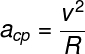cp – centripetal acceleration (m/s²)

Generally, it is necessary to analyze the forces acting on the body so that it is possible to establish which is the resultant force responsible for producing the circular motion. When cars turn, for example, it is the frictional force between the tires and the ground that changes the direction of their movement; in the case of planets orbiting the Sun, the role of the centripetal force is played by the gravitational force .

Therefore, there are different configurations of centripetal force, and therefore it is necessary to realize that each problem may have a different resultant centripetal force . So in this article, we’ll explore some common centripetal cases, and then come up with some solved exercises.

## Centripetal force and frictional force

This situation is used to solve exercises that deal with vehicles, such as cars and motorcycles, that enter curves with a certain speed. To solve them, we say that the centripetal force is the friction force between the tires and the ground , note: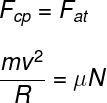μ – coefficient of friction

N – normal force (N)

In the case where we want to determine what the maximum speed should be, or even the minimum radius of the curve so that a moving body does not skid , it is necessary to use the coefficient of static friction , since, in this situation, the vehicle tires only roll across the floor without slipping.

## Centripetal force on speed bumps and depressions

When a vehicle passes over a speed bump, or even through a depression in the road, a centripetal force acts on it, so that it can make the turn. This centripetal force can be perceived by the occupants of the vehicle as “butterflies in the stomach” , since the inertia of our internal organs tends to keep them in their state of motion when we enter curves.

The next figure shows how we calculate the resultant force when a piece of furniture passes over a spine, check it out:

Now, we show the situation where a mobile passes over a depression, observe how we calculate the centripetal force:

## Centripetal force and traction

When a body is attached to a wire , string , or cable, and is set to rotate, the centripetal force is exerted by the pulling force , as when the body rotates in a circular path, supported on a horizontal plane. In the case of a pendulum, in turn, the centripetal force is determined by the resultant of the weight force and the pulling force.

## Centripetal force and gravitational force

Since the gravitational force always points in the radial direction, it is able to play the role of a centripetal force. In the case where there is exclusively the gravitational force on a body, we say that the centripetal force is equal to the gravitational force :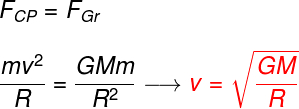G – constant of universal gravitation

M and m – gravitational masses

The result obtained in the calculation allows us to know the orbital speed, that is, the speed with which a planet revolves around the Sun, or even the speed with which a satellite orbits the Earth, for example.

## Centripetal force on the globe of death

The Globe of Death is a circus attraction in which motorcyclists make complete turns, inside a large metallic globe, without falling, thanks to its great speed and the effect of its inertia. Next, we will show a scheme of how centripetal force is calculated when the motorcycle is at the bottom of the globe of death and when it is on the verge of falling, but is still able to complete the circle of the globe.

Now, we bring a scheme of how the normal force is calculated for cases where the motorcycle is at the bottom of the globe of death; at the top of the globe of death, but with speed above the minimum speed necessary to cross it; and, finally, at the top of the globe of death, the minimum speed so that it does not fall: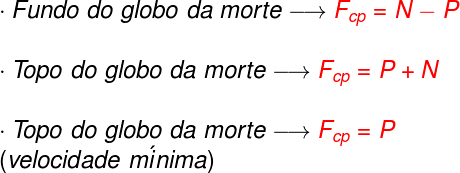## Solved exercises on centripetal force

Question 1) A car of mass 1000 kg enters, at 30 m/s, in a curve of radius equal to 300 m, contained in a horizontal surface. Determine the magnitude of the coefficient of static friction between the car’s tires and the asphalt so that this vehicle does not skid.

a) 0.5

b) 3

c) 0.3

d) 0.2

e) 0.8

Template : Letter C

Resolution :

To make this calculation, it is necessary to note that the friction force between the tires and the ground plays the role of the centripetal force. After that, it is necessary for us to remember the relationship between the normal force and the weight: these forces have the same intensity when on flat surfaces, so we just need to do the following calculation: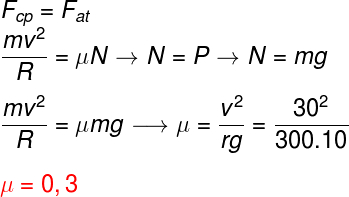Question 2) A 50 g toy car is attached to a 0.05 m long rope and rotated around a nail that is fixed to a perfectly horizontal surface. Knowing that the speed of the cart is 0.1 m/s, determine the magnitude of the pulling force that is exerted by the rope, disregarding the action of any dissipative forces.

a) 1.0.10 -2 N

b) 5.0.10 -3 N

c) 1.0.10 -4 N

d) 6.0.10 -1 N

e) 1.0.10 -3 N

Template: Letter A

Resolution :

In this exercise, the centripetal force is performed by the pulling force exerted by the rope, so we just have to do the following calculation: